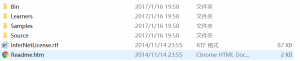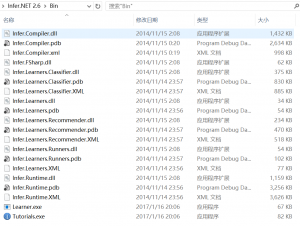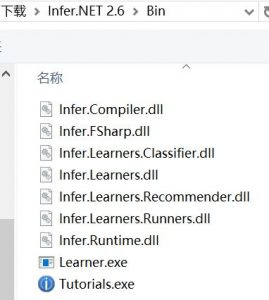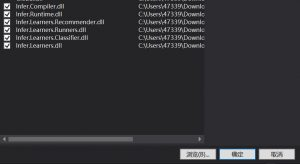# Microsoft Infer.NET使用手册1

### Microsoft Infer.NET使用手册1（顺带一提，NuGet程序包里也有Infer.NET，但是那个不提供learners的所有API，所以建议还是从官网下载手动导入）

[code lang=”CSharp”]

public string name;     //学生的名字
public Vector c_m_s_e;   //语数英科成绩, Vector需要引用MicrosoftResearch.Infer.Maths。
[/code]

[code lang=”CSharp”]
public Student(string Name, Vector FourSubjects)
{
name = Name;
c_m_s_e = FourSubjects;
}
[/code]

[code lang=”CSharp”]
public static Vector getGrades(double chinese, double english, double math, double science)
{
return Vector.FromArray(chinese,english,math,science);
}
[/code]

Student类，完成。

[code lang=”CSharp”]
using MicrosoftResearch.Infer.Learners.Mappings;
using MicrosoftResearch.Infer.Maths;
[/code]

IClassifierMapping作为较为简单的一种分类器映射，它具有4个需要实现的方法，这四个方法的作用就是让分类器获取实例，特征，以及标签的。这四个方法分别是：

[code lang=”CSharp”]
IEnumerable<TLabel>; GetClassLabels(TInstanceSource instanceSource = default(TInstanceSource), TLabelSource labelSource = default(TLabelSource));
[/code]

[code lang=”CSharp”]
TFeatures GetFeatures(TInstance instance, TInstanceSource instanceSource = default(TInstanceSource));
[/code]

[code lang=”CSharp”]
IEnumerable<TInstance>; GetInstances(TInstanceSource instanceSource);
[/code]

[code lang=”CSharp”]
TLabel GetLabel(TInstance instance, TInstanceSource instanceSource = default(TInstanceSource), TLabelSource labelSource = default(TLabelSource));
[/code]

[code lang=”CSharp”]
class StudentClassifier : IClassifierMapping<IList<Student>, Student, IList<bool>, bool, Vector>
{
public IEnumerable<bool> GetClassLabels(IList<Student> instanceSource = null, IList<bool> labelSource = null)
{
return new bool[] { true, false };
}

public Vector GetFeatures(Student instance, IList<Student> instanceSource = null)
{
return instance.c_m_s_e;
}

public IEnumerable<Student> GetInstances(IList<Student> instanceSource)
{
return instanceSource;
}

public bool GetLabel(Student instance, IList<Student> instanceSource = null, IList<bool> labelSource = null)
{
return instance.good;
}
}
[/code]

[code lang=”CSharp”]
using MicrosoftResearch.Infer.Learners;
[/code]

[code lang=”CSharp”]
StudentClassifier classifier = new StudentClassifier();
[/code]

[code lang=”CSharp”]
var classMachine = BayesPointMachineClassifier.CreateBinaryClassifier(classifier);
[/code]

[code lang=”CSharp”]
List<Student> students = new List&lt;Student&gt;()
};

List<bool> labels = new List<bool>() { true, true, false };
[/code]

[code lang=”CSharp”]
classMachine.Train(students, labels);
[/code]

[code lang=”CSharp”]
Student someone = new Student("somebody", Student.getGrades(90, 80, 92, 70));
bool isGoodStudent = classMachine.Predict(someone);
System.Console.WriteLine(isGoodStudent);
[/code]

program类中所以代码应该是这样的：

[code lang=”CSharp”]
using System.Collections.Generic;
using MicrosoftResearch.Infer.Learners;
namespace InfernetTutorial
{
class Program
{
static void Main(string[] args)
{
StudentClassifier classifier = new StudentClassifier();
var classMachine = BayesPointMachineClassifier.CreateBinaryClassifier(classifier);
List<Student> students = new List&lt;Student&gt;()
};

List&lt;bool&gt; labels = new List<bool>() { true, true, false };
classMachine.Train(students, labels);
Student someone = new Student("somebody", Student.getGrades(90, 80, 92, 70));
bool isGoodStudent = classMachine.Predict(someone);
System.Console.WriteLine(isGoodStudent);

}
}
}
[/code]

P.S.:其实经本人亲自测试，这里提供给分类器的数据并不好，大家可以再尝试其他的方式来玩玩这个东西。